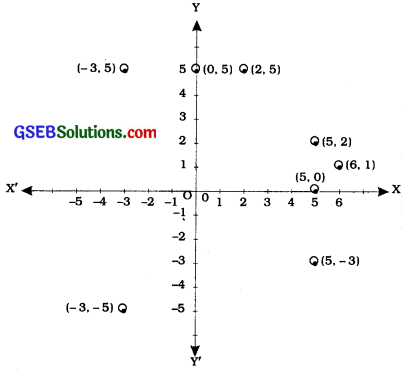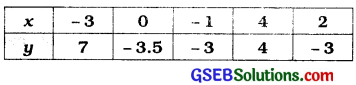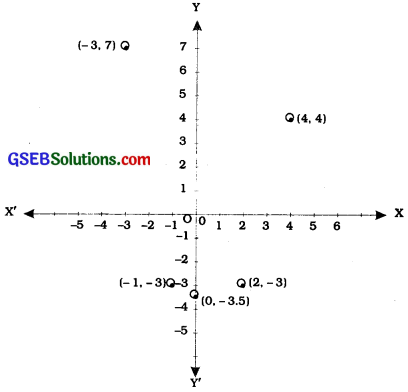# GSEB Class 9 Maths Notes Chapter 3 Introduction to Euclid’s Geometry

This GSEB Class 9 Maths Notes Chapter 3 Introduction to Euclid’s Geometry covers all the important topics and concepts as mentioned in the chapter.

## Introduction to Euclid’s Geometry Class 9 GSEB Notes

Number Line:
Every real number is represented by a unique point on the number line and every point on the number line represents a unique real number.
Thus, every point on the number line is the graph or location of a single real number on a line.
To represent an ordered pair of real numbers, we have to represent it in a plane with the help of two number lines.

Cartesian coordinate system:
Cartesian plane:
Draw two perpendicular lines in the plane; one horizontal and the other vertical. Their point of intersection O is called the origin. The horizontal line is called the x-axis and the vertical line is called the y-axis. Both the axes are called the coordinate axes. The plane is called the coordinate plane.

• There is one-one correspondence between a line and the collection of real numbers (R). Hence, the points on the x-axis as well as the points on the y-axis correspond to real numbers.
• The plane is called the Coordinate plane or the Cartesian plane or the xy-plane.

As we know, positive numbers lie to the right side of O and negative numbers lie to the left side of O on a number line. Similarly, on the x-axis, positive numbers lie to the right of O, i.e., on ray OX and negative numbers lie to the left of O, i.e., on ray OX’. In the same manner, on the y-axis, positive numbers lie on the upper side of O, i.e., on ray OY and negative numbers lie on the lower side of O, i.e., on ray OY’. OX and OY are called the positive directions of the x-axis and the y-axis respectively. Similarly, OX’ and OY’ are called the negative directions of the x-axis and the y-axis respectively.

One-one correspondence between the plane and ordered pair of real numbers:
Corresponding to each ordered pair (x, y), where x and y are real numbers, there is a unique point P in the plane.
Corresponding to each point P in the plane, there is a unique ordered pair (x, y), where x and y are real numbers.
If point P in the plane and ordered pair (x, y) correspond to each other, it is denoted by writing P(x, y).
P is called the graph of (x, y) in the plane and (x, y) are called the coordinates of point R x is called the x-coordinate or abscissa of P and y is called the y-coordinate or ordinate of P Just like P (x, y) is also called a point of the plane.

• The x-coordinate of a point is its perpendicular distance from the y-axis measured along the x-axis (positive along the positive direction of the x-axis and negative along the negative direction of the x-axis).
• The y-coordinate of a point is its perpendicular distance from the x-axis measured along the y-axis (positive along the positive direction of the y-axis and negative along the negative direction of the y-axis).

Example 1: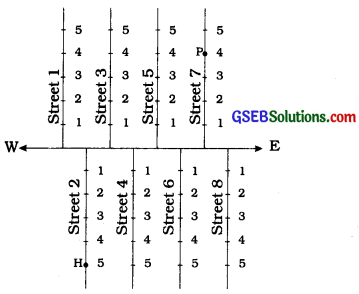In the given figure there is a main road running in the East-West direction and streets with numbering from West to East. Also, on each street, house numbers are marked. To look for a friend’s house here, is it enough to know only one reference point? For instance, if we only know that she lives on street 2, will we be able to find her house easily?
Not as easily as when we know two pieces of information about it, namely, the number of the street on which it is situated, and the house number. If we want to reach the house which is situated in the 2nd street and has the number 5, first of all we would identify the 2nd street and then the house numbered 5 on it. In the given figure, H shows the location of the house. Similarly, P shows the location of the house corresponding to street number 7 and house number 4.

Example 2:
Suppose you put a dot on a sheet of paper [fig. (a)]. If we ask you to tell us the position of the dot on the paper, how will you do this? Perhaps you will try in some such manner: “The dot is in the upper half of the paper”, or “It is near the left edge of the paper”, or “It is very near the left hand upper corner of the sheet”. Do any of these statements fix the position of the dot precisely? No! But, if you say “The dot is nearly 5 cm away from the left edge of the paper”, it helps to give some idea but still does not fix the position of the dot. A little thought might enable you to say that the dot is also at a distance of 9 cm above the bottom line. We now know exactly where the dot is!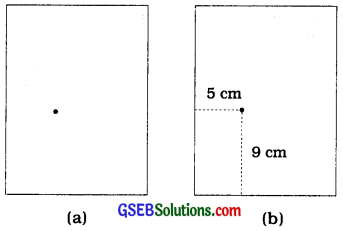For this purpose, we fixed the position of the dot by specifying its distances from two . fixed lines, the left edge of the paper and the bottom line of the paper [fig. (b)]. In other words, we need two independent informations for finding the position of the dot.In the Cartesian coordinate system, the perpendicular axes divide the plane in four parts. Each part Is called a quadrant.

 Quadrant Part x-coordinate y-coordinate 1. FIrst quadrant Interior of ∠XOY + ve + ve 2. Second quadrant Interior of ∠YOX’ – ve + ve 3. Third quadrant Interior of ∠X’OY’ – ve – ve 4. Fourth quadrant Interior of ∠YOX + ve – ve

Observe the graph below: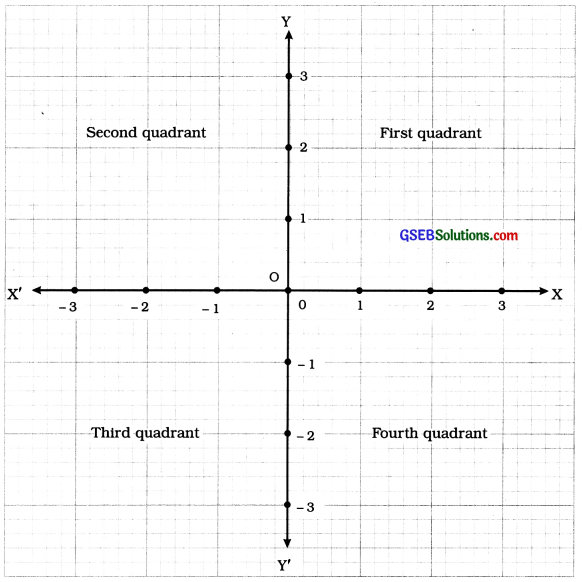Points on the axes:

• If the y-coordtnate of a point is O. that point lies on the x-axis arid Its coordinates are of the form (a. 0).
• If the x-coordlnate of a point Is O. that point lies on the y-axis and its coordinates are of the form (O. b).
• The coordinates of the origin are (O. O).

To find the coordinates of a point lying In any quadrant, perpendiculars are drawn from that point to both the axes. The number corresponding to the foot of perpendicular on the x-axis gives the x-coordlnate or the abscissa of the point and the number corresponding to the foot of perpendicular on the y-axis gives the y-coordInate or the ordinate of the point.

Example 1:
See the figure given below and complete the following statements: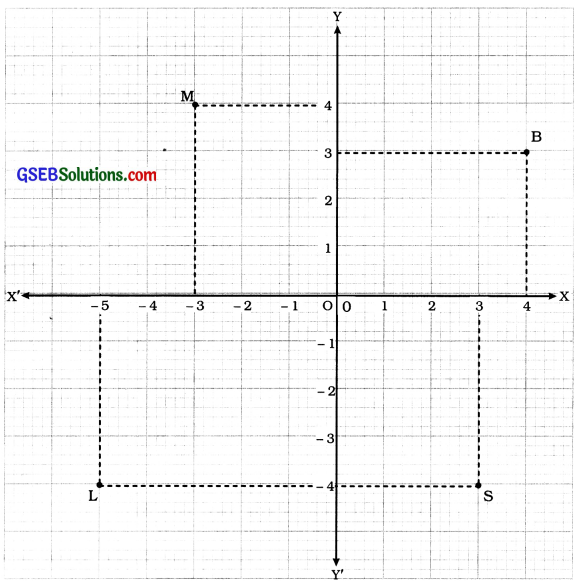(i) The abscissa and the ordinate of the point B are ______ and ______ respectively. Hence, the coordinates of B are (______, ______)
Since the distance of the point B from the y-axis is 4 units, the x-coordinate or abscissa of the point B is 4. The distance of the point B from the x-axis is 3 units; therefore, the y-coordinate, i.e., the ordinate of the point B is 3. Hence, the coordinates of the point B are
(4, 3).

(ii) The x-coordinate and the y-coordinate of the point M are ______ and ______ respectively. Hence, the coordinates of M are (______, ______).
As in (i) above:
The x-coordinate and the y-coordinate of the point M are -3 and 4 respectively. Hence, the coordinates of the point M are (-3, 4).

(iii) The x-coordinate and the y-coordinate of the point L are ______ and ______ respectively. Hence, the coordinates of L are (______, ______).
The x-coordinate and the y-coordinate of the point L are -5 and -4 respectively. Hence, the coordinates of the point L are (-5, -4).

(iv) The x-coordinate and the y-coordinate of the point S are ______ and ______ respectively. Hence, the coordinates of S are (______, ______)
The x-coordinate and the y-coordinate of the point S are 3 and -4 respectively. Hence, the coordinates of the point S are (3, -4).

Example 2:
Write the coordinates of the points marked on the axes in the given figure: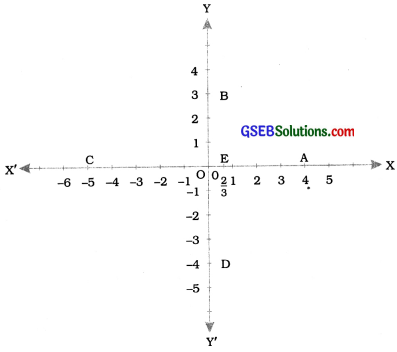(i) The point A is at a distance of + 4 units from the y-axis and at a distance zero from the x-axis. Therefore, the x-coordinate of A is 4 and the y-coordinate 1 0. Hence, the coordinates of A are (4, 0)

(ii) The coordinates of B are (0, 3). Why?
Point B Is at a distance zero from the y-axis and at a distance + 3 from the x-axis. Hence, the coordinates of B are (0, 3).

(iii) The coordinates of C are (-5, 0). Why?
Point C is at a dIstance -5 from the y-axis and at a distance zero from the x-axis. Hence, the coordinates of C are (-5. 0)

(iv) The coordinates of D are (0, -4). Why?
Point D is at a distance zero from the y-axis and at a distance -4 from the x-axis. Hence, the coordinates of D are (0, -4).

(v)The coordinates of E are ($$\frac{2}{3}$$, o). Why?
Point E Is at a distance $$\frac{2}{3}$$ from the y-axis and at a distance zero from the x-axis. Hence, the coordinates of E are ($$\frac{2}{3}$$, 0).

Plotting a point in the plane:
Suppose we want to plot the point (a, b) In the Cartesian plane. First of all find point M on the x-axis corresponding to a and draw a perpendicular to the x-axis through M. Similarly, find point N on the y-axis corresponding to b and draw a perpendicular to the y-axis through N. The unique point P of the Intersection of these perpendiculars is the point In the plane corresponding to (a, b). P Is the graph of (a, b) in the Cartesian plane. Point with coordinates (x, O) lies on the x-axis and point with. coordinates (0, y) 11es on the y-axis.

Note:

• After enough practice, we can plot point P (a, b) without drawing the perpendiculars.
• Ordered pairs (x. y) and (a, b) of real numbers are equal if and only If x = a and y = b.

Example 1:
Locate the points (5, 0), (0, 5), (2, 5), (5, 2), (-3, 5), (-3, -5), (5, -3) and (6, 1) in the Cartesian plane.